# afex_plot: Publication Ready Plots for Experimental Designs

#### 2018-09-24

afex_plot() visualizes results from factorial experiments combining estimated marginal means and uncertainties associated with the estimated means in the foreground with a depiction of the raw data in the background. Currently, afex_plots() supports the following models:

• ANOVAs estimated with aov_car(), aov_ez(), or aov_4() (i.e., objects of class "afex_aov")
• Linear mixed models estimated with mixed() (i.e., objects of class "mixed")
• Linear mixed models estimated with lme4::lmer (i.e., objects of class "merMod")

This document provides an overview of the plots possible with afex_plot(). It does so mostly using the afex_plot() examples, see ?afex_plot. We begin by loading afex and ggplot2 which is the package afex_plot() uses for plotting. Loading ggplot2 explicitly is not strictly necessary, but makes the following code nicer. Otherwise, we would need to prepend each call to a function from ggplot2 needed for customization with ggplot2:: (as is done in the examples in ?afex_plot).

We also load the cowplot package (introduction) which makes combining plots (with functions plot_grid() and legend()) very easy. However, loading cowplot sets a different theme for ggplot2 plots than the default grey one. Although I am not a big fan of the default theme with its grey background, we reset the theme globally using theme_set(theme_grey()) to start with the default behavior if cowplot it not attached. Note that cowplot also has the cool draw_plot() function which allows embedding plots within other plots.

We furthermore will need the following packages, however, we will not attach them directly, but only call a few selected functions using the package::function notation.

library("afex")
library("ggplot2")
library("cowplot")
theme_set(theme_grey())

# Two-Way Within-Subjects ANOVA

We begin with a two-way within-subjects ANOVA using synthetic data from Maxwell and Delaney (2004, p. 547). The data are hypothetical reaction times from a 2 x 3 Perceptual Experiment with factors angle with 3 levels and factor noise with 2 levels (see ?md_12.1 for a longer description). We first load the data and then fit the corresponding ANOVA.

data(md_12.1)
(aw <- aov_ez("id", "rt", md_12.1, within = c("angle", "noise")))
## Anova Table (Type 3 tests)
##
## Response: rt
##        Effect          df     MSE         F ges p.value
## 1       angle 1.92, 17.31 3702.02 40.72 *** .39  <.0001
## 2       noise        1, 9 8460.00 33.77 *** .39   .0003
## 3 angle:noise 1.81, 16.27 1283.22 45.31 *** .19  <.0001
## ---
## Signif. codes:  0 '***' 0.001 '**' 0.01 '*' 0.05 '+' 0.1 ' ' 1
##
## Sphericity correction method: GG

The ANOVA shows that both, the two main effect as well as the interaction, are significant. We therefore inspect the pattern underlying the interaction. There exist two different ways of plotting a 2-way interaction. Either of the two variables can be depicted on the x-axis. And before having looked at both cases, it is often not clear which visualization of the interaction is more instructive. Consequently, we plot both next to each other. For this we simply need to exchange which variable is the x factor and which is the trace factor. We then use plot_grid() to plot them next to each other.

## Basic Plot

p_an <- afex_plot(aw, x = "angle", trace = "noise") 
## Warning: Panel(s) show within-subjects factors, but not within-subjects error bars.
## For within-subjects error bars use: error = "within"
p_na <- afex_plot(aw, x = "noise", trace = "angle")
## Warning: Panel(s) show within-subjects factors, but not within-subjects error bars.
## For within-subjects error bars use: error = "within"
plot_grid(p_an, p_na)  ## try adding: labels = "AUTO"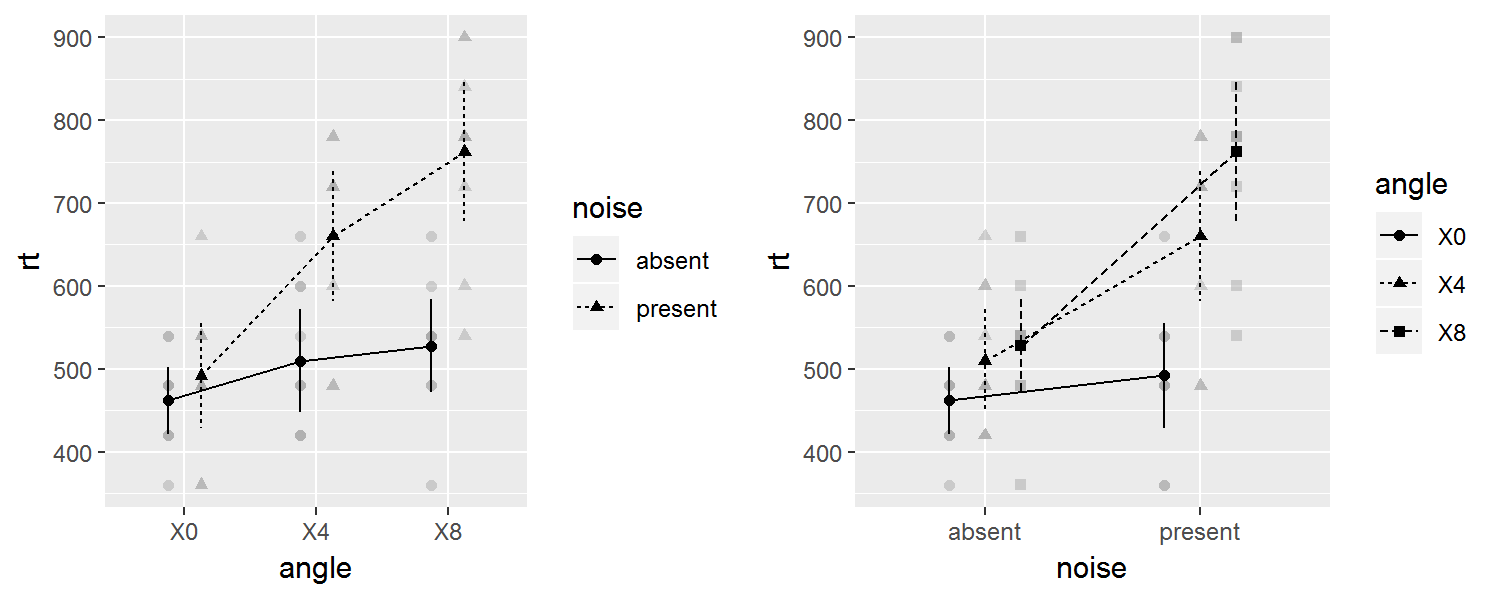Before we can even take a look at the plot, we notice that creating the plots has produced two warnings. These warnings complain that the plots depict within-subject factors, but do not use within-subject error bars. However, the warnings also tell us the solution (i.e., adding error = "within"), which we will do in the following. The help page ?afex_plot contains more information on which type of error bars are appropriate in which situation and how to interpret different type of error bars. For ANOVAs, afex_plot() will emit warnings if it thinks the error bars are not appropriate for the chosen factors.

Comparing both plots, my impression is that the plot with angle on the x-axis tells the clearer story. We can see that when noise is absent there is hardly any effect of the increase of angle. However, if noise is present an increasing angle clearly leads to increased RTs. We therefore use this plot in the following.

## Exploring Graphical Options and Themes

We now produce a new variant of the left plot using more appropriate error bars and change several other graphical details which make the plot publication ready. We use the ability of afex_plot() to rename the factor levels (for technical reasons the ANOVA functions in afex transform all factor levels to proper R variable names using make.names() which changed the labels from e.g., 4 to X4) and the title of the legend. We also change the labels on the x and y axis.

p_an <- afex_plot(aw, x = "angle", trace = "noise", error = "within",
factor_levels = list(angle = c("0°", "4°", "8°"),
noise = c("Absent", "Present")),
legend_title = "Noise") +
labs(y = "RTs (in ms)", x = "Angle (in degrees)")

As said above, I am not a big fan of the default grey theme of ggplot2 plots. Consequently, we compare a number of different themes for this plot in the following. For all but ggpubr::theme_pubr(), we also move the legend to the bottom as this better allows the plot to cover only a single column in a two-column layout. ggpubr::theme_pubr() automatically plots the legend on top.

plot_grid(
p_an + theme_bw() + theme(legend.position="bottom"),
p_an + theme_light() + theme(legend.position="bottom"),
p_an + theme_minimal() + theme(legend.position="bottom"),
p_an + jtools::theme_apa() + theme(legend.position="bottom"),
p_an + ggpubr::theme_pubr(),
p_an + theme_cowplot() + theme(legend.position="bottom"),
labels = "AUTO"
)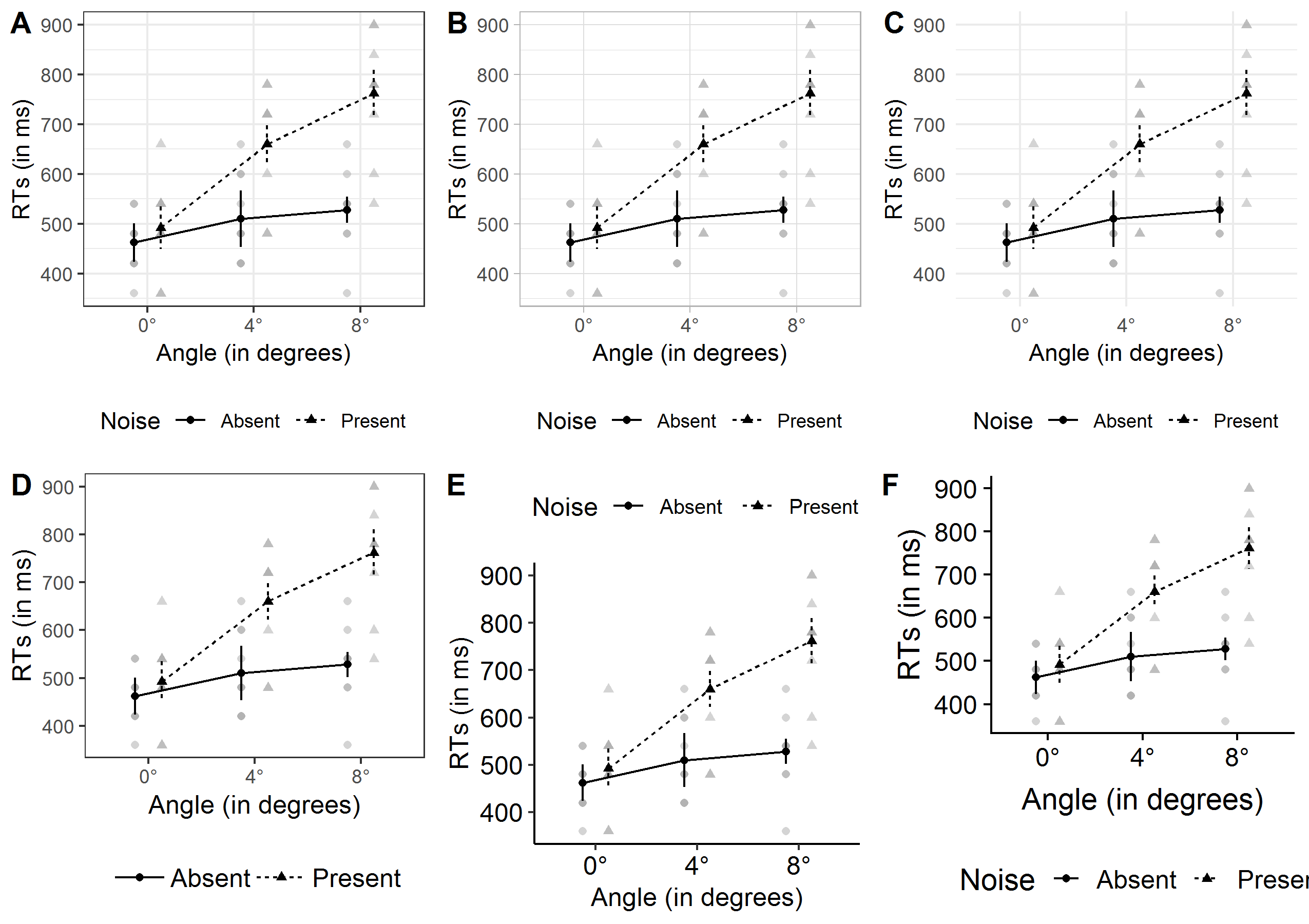The first row, panels A to C, shows themes coming with ggplot2 and the second row, panels D to F, shows themes from additional packages. In my opinion all of these plots are an improvement above the default grey theme. For the themes coming with ggplot2, I really like that those shown here have a reference grid in the background. This often makes it easier to judge the actual values the shown data points have. I know that many people find this distracting, so many of the contributed themes do not have this grid. One thing I really like about the last two themes is that they per default use larger font sizes for the axes labels. One way to achieve something similar for most themes is to change base_size.

One general criticism I have with the current plots is that they show too many values on the y-axis. In the following I plot one more variant of this plot in which we change this to three values on the y-axis. We also increase the axes labels and remove the vertical grid lines.

p_an +
scale_y_continuous(breaks=seq(400, 900, length.out = 3)) +
theme_bw(base_size = 15) +
theme(legend.position="bottom",
panel.grid.major.x = element_blank())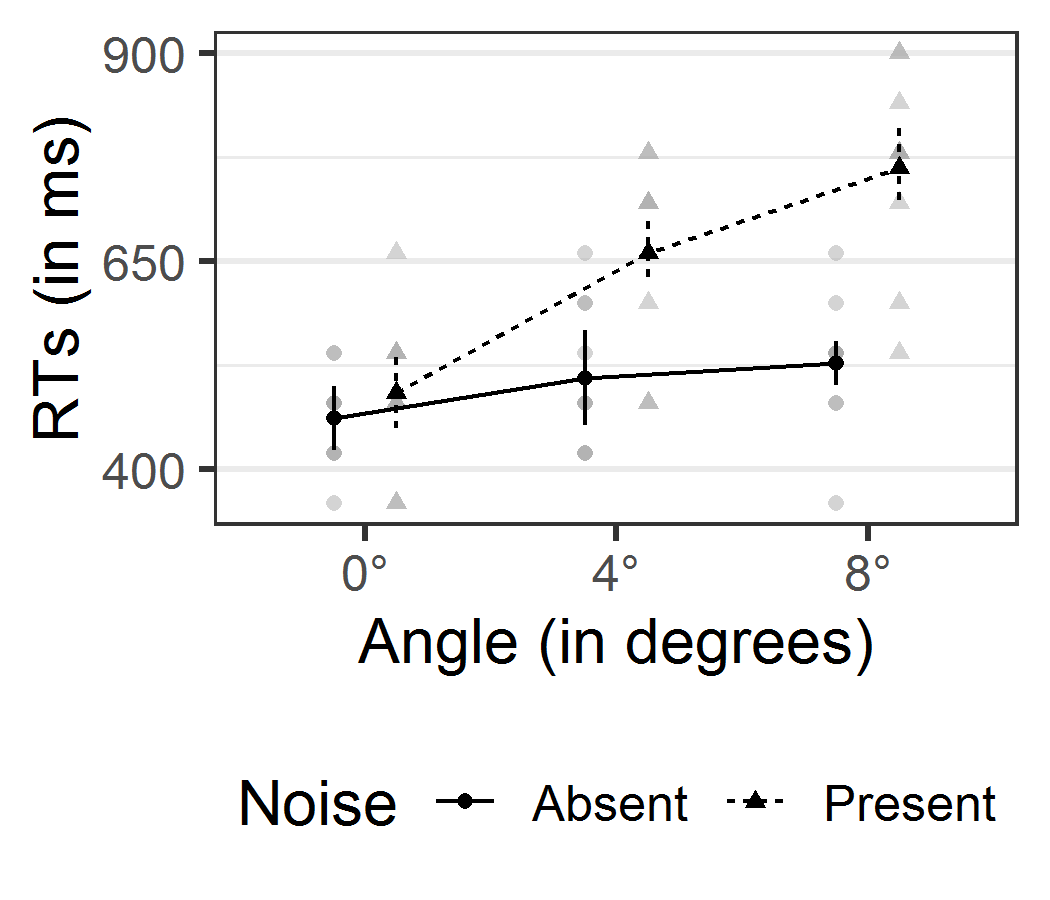We can also set this theme for the reminder of the R session with theme_set().

theme_set(theme_bw(base_size = 15) +
theme(legend.position="bottom",
panel.grid.major.x = element_blank()))

## Saving Plots and Plot Sizes

To get our plot into a publication, we need to export it as a graphics file. I would generally advise against exporting plots via the RStudio interface as this is not reproducible. Instead I would use some of the following functions which save the document in the current working directory. Note that following Elsevier guidelines, a single column figure should have a width of 9 cm (~ 3 inch) and a two column figure should have a width of 19 cm (~ 7.5 inch).

For Word or similar documents I would export the plot as a png (never jpg):

ggsave("my_plot.png", device = "png",
width = 9, height = 8, units = "cm",
dpi = 600) ## the higher the dpi, the better the resolution

For LaTeX I would export as pdf:

ggsave("my_plot.pdf", device = "pdf",
width = 9, height = 8, units = "cm") 

## Data in the Background

afex_plot() per default plots the raw data in the background. It does so using an alpha blending of 0.5. Thus, overlapping points appear darker. Examples of this can be seen in the previous graphs where some data points in the background appear clearly darker than others. The darker points indicate values for which several data points lie exactly on top of each other.

afex_plot() provides the possibility to change or alter the graphical primitive, called geom in ggplot2 parlance, used for plotting the points in the background. This offers a vast array of options for handling overlapping points or, more generally, how to display the raw data in the background. I show some of these examples in the following.

The first two variants display only points, whereas the remaining ones use different visualizations of the raw data. Note that depending on the specific variant we change a few further plot options to obtain a visually pleasing result. For example, the dodge argument controls the spread of points belonging to different levels of the trace factor at each x-axis position.

1. Add jitter on the y-axis to points which avoids perfect overlap.
2. Display points using a bee swarm plot, which displaces overlapping points on the x-axis: ggbeeswarm::geom_beeswarm
3. Violin plot: geom_violin
4. Box plot: geom_boxplot
5. Combine box plot with jittered points: ggpol::geom_boxjitter
p1 <- afex_plot(aw, x = "noise", trace = "angle", error = "within", dodge = 0.3,
data_arg = list(
position =
ggplot2::position_jitterdodge(
jitter.width = 0,
jitter.height = 10,
dodge.width = 0.3  ## needs to be same as dodge
),
color = "darkgrey"))
p2 <- afex_plot(aw, x = "noise", trace = "angle", error = "within", dodge = 0.5,
data_geom = ggbeeswarm::geom_beeswarm,
data_arg = list(
dodge.width = 0.5,  ## needs to be same as dodge
cex = 0.8,
color = "darkgrey"))
p3 <- afex_plot(aw, x = "noise", trace = "angle", error = "within",
data_geom = ggplot2::geom_violin,
data_arg = list(width = 0.5))
p4 <- afex_plot(aw, x = "noise", trace = "angle", error = "within",
data_geom = ggplot2::geom_boxplot,
data_arg = list(width = 0.3))
p5 <- afex_plot(aw, x = "noise", trace = "angle", error = "within", dodge = 0.7,
data_geom = ggpol::geom_boxjitter,
data_arg = list(
width = 0.5,
jitter.width = 0,
jitter.height = 10,
outlier.intersect = TRUE),
point_arg = list(size = 2.5),
error_arg = list(size = 1.5, width = 0))
plot_grid(p1, p2, p3, p4, p5, ncol = 2, labels = 1:5)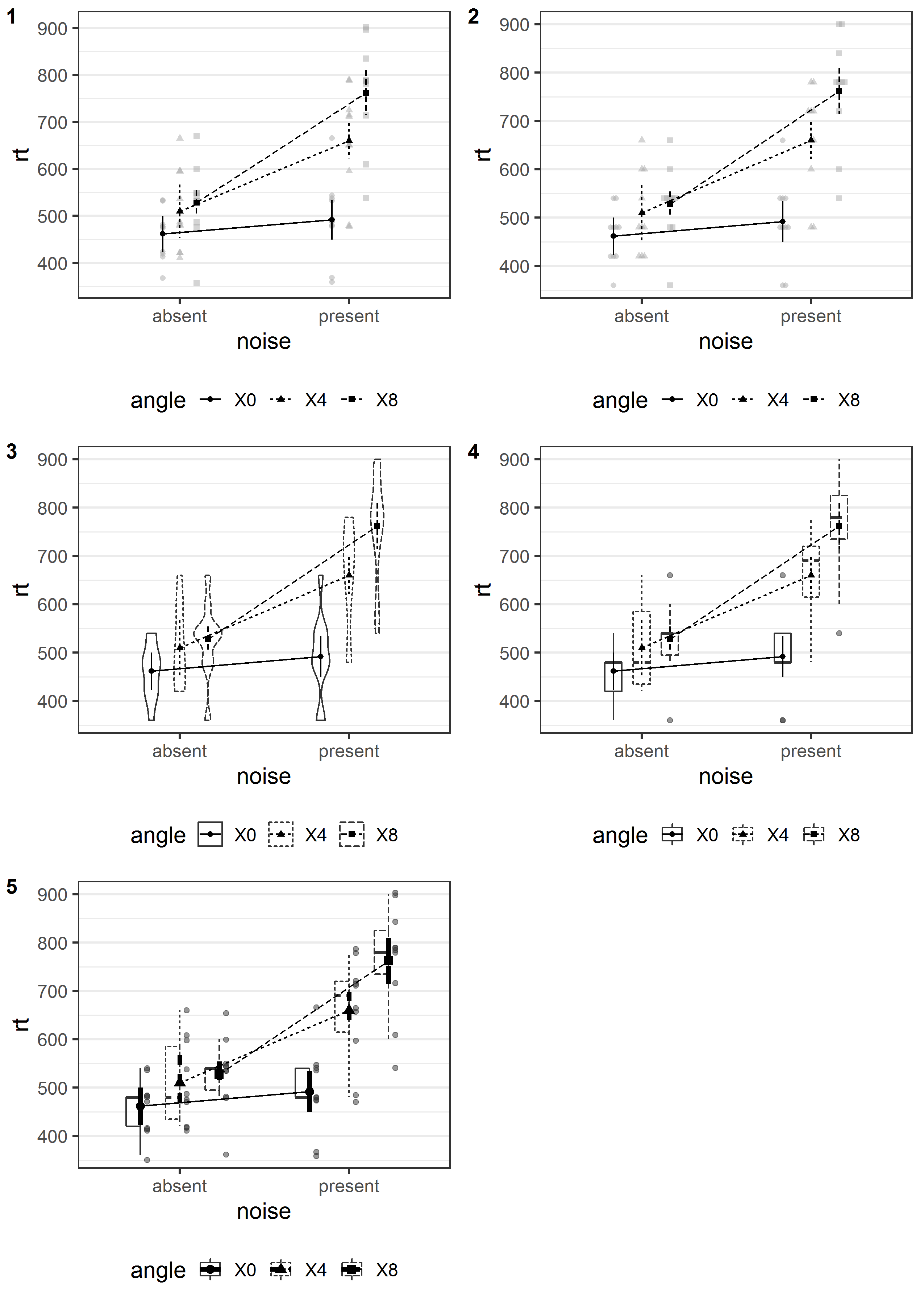So far, all plots were shown in black and white only. However, it is easy to include color. We do so for plots 2 to 5 from above. To achieve this, we have to change the value of the mapping argument.

p2 <- afex_plot(aw, x = "noise", trace = "angle", error = "within", dodge = 0.5,
mapping = c("shape", "color"),
data_geom = ggbeeswarm::geom_beeswarm,
data_arg = list(
dodge.width = 0.5,  ## needs to be same as dodge
cex = 0.8))
p3 <- afex_plot(aw, x = "noise", trace = "angle", error = "within",
mapping = c("linetype", "shape", "fill"),
data_geom = ggplot2::geom_violin,
data_arg = list(width = 0.5))
p4 <- afex_plot(aw, x = "noise", trace = "angle", error = "within",
mapping = c("shape", "fill"),
data_geom = ggplot2::geom_boxplot,
data_arg = list(width = 0.3))
p5 <- afex_plot(aw, x = "noise", trace = "angle", error = "within", dodge = 0.7,
mapping = c("shape", "fill"),
data_geom = ggpol::geom_boxjitter,
data_arg = list(
width = 0.5,
jitter.width = 0,
jitter.height = 10,
outlier.intersect = TRUE),
point_arg = list(size = 2.5),
line_arg = list(linetype = 0),
error_arg = list(size = 1.5, width = 0))
plot_grid(p2, p3, p4, p5, ncol = 2, labels = 2:5)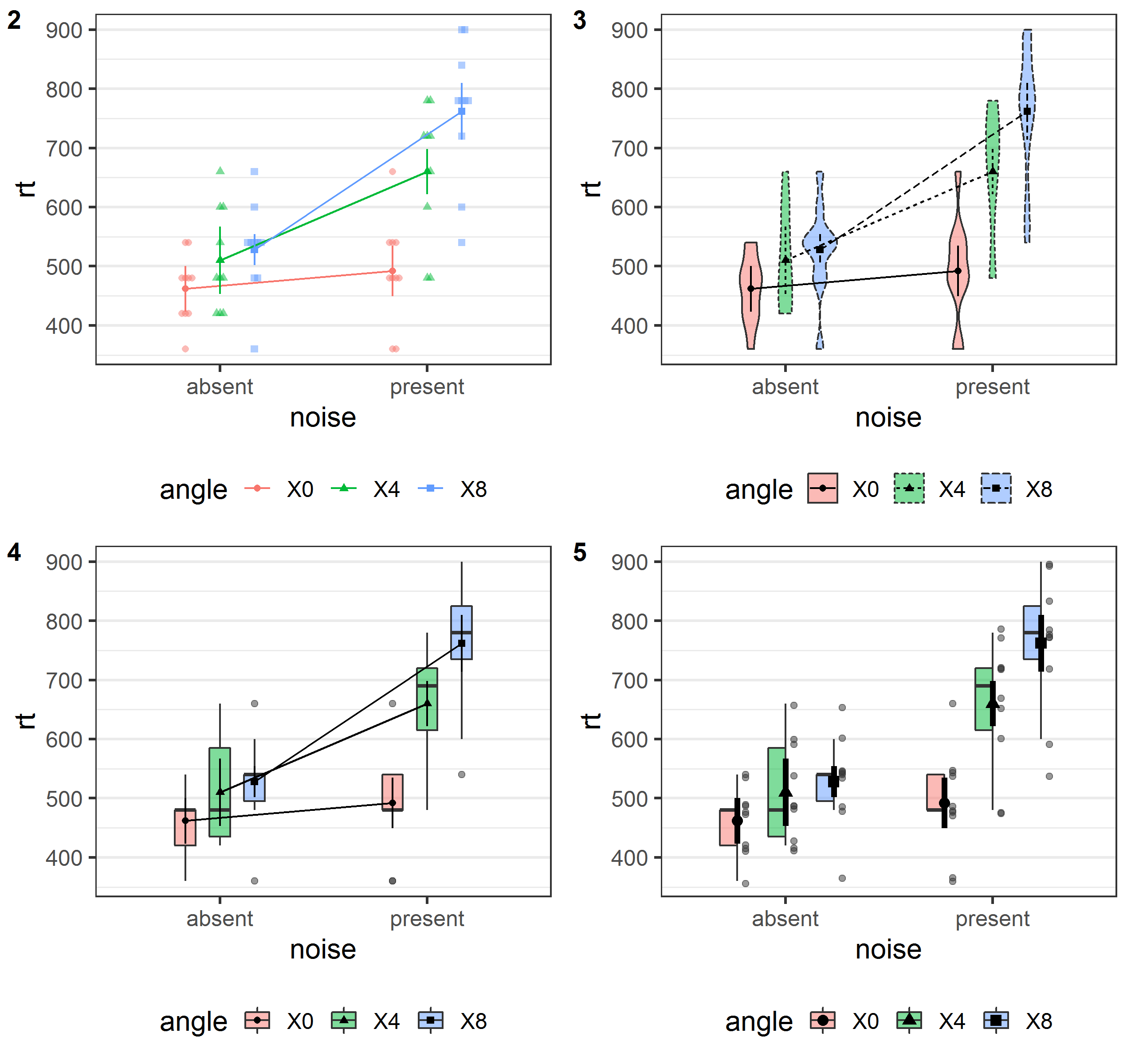## One-way Plots Without Trace Factor

If afex_plot is called without a trace factor, a one-way plot is created. We can customize this plot in very much the same way. Per default a one-way plot contains a legend if mapping is not empty (i.e., ""). We show this legend for the left plot, but suppress it for the right one.

po1 <- afex_plot(aw, x = "angle", mapping = "color", error = "within",
data_arg = list(),
point_arg = list(size = 2.5),
error_arg = list(size = 1.5, width = 0.05))
po2 <- afex_plot(aw, x = "angle", error = "within",
data_geom = ggpol::geom_boxjitter,
mapping = "fill", data_alpha = 0.7,
data_arg = list(
width = 0.6,
jitter.width = 0.05,
jitter.height = 10,
outlier.intersect = TRUE
),
point_arg = list(size = 2.5),
error_arg = list(size = 1.5, width = 0.05)) +
theme(legend.position="none")
plot_grid(po1, po2)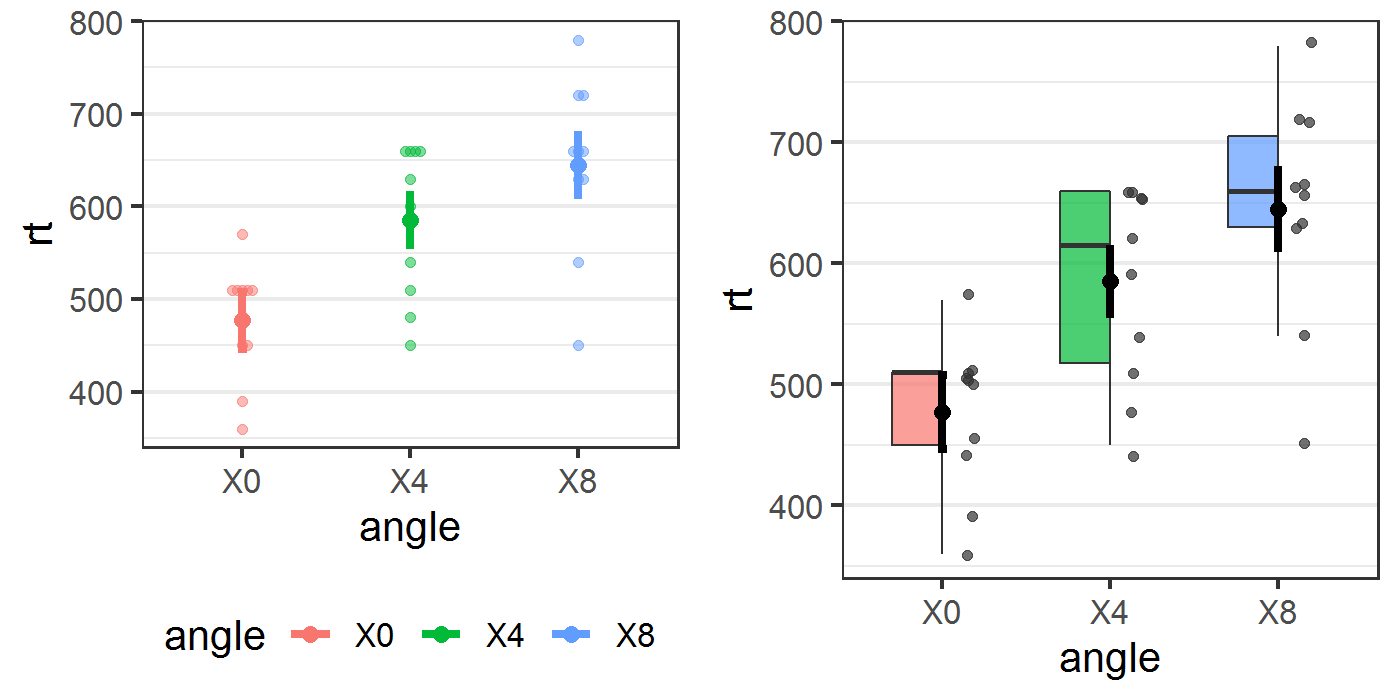One-way plots can also be split across different panels by specifying a panel factor:

afex_plot(aw, x = "angle", panel = "noise", error = "within",
data_geom = ggpol::geom_boxjitter,
mapping = "fill", data_alpha = 0.7,
data_arg = list(
width = 0.6,
jitter.width = 0.05,
jitter.height = 10,
outlier.intersect = TRUE
),
point_arg = list(size = 2.5),
error_arg = list(size = 1.5, width = 0.05)) +
theme(legend.position="none")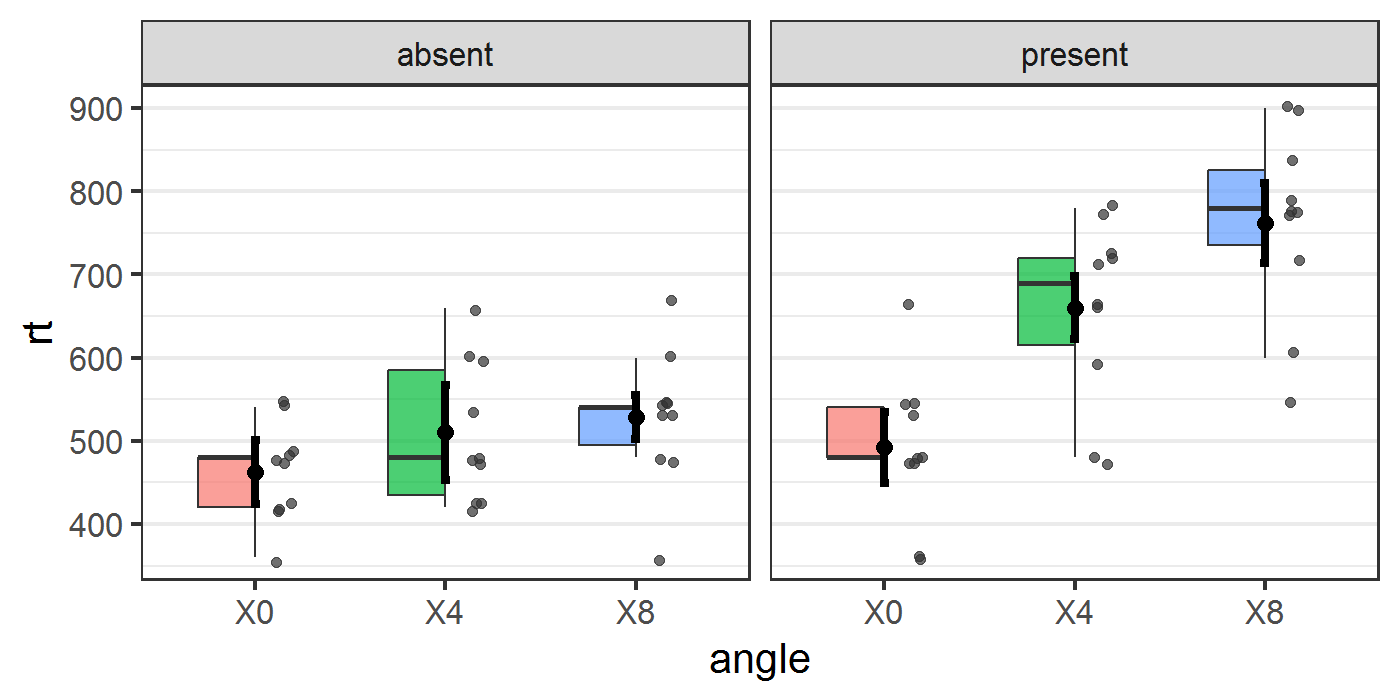# 3-Way Mixed Model

## Data and Model

To exemplify the support for linear mixed models, we will use the data from Freeman and colleagues also discussed in the mixed model vignette. These data are lexical decision and word naming latencies for 300 words and 300 nonwords from 45 participants presented in Freeman et al. (2010). The dependent variable we are interested in is log RTs. Here, we are only looking at a reduced design with factors task (between participants, but within items), stimulus, and frequency (within participants, but between items), for a total of almost 13,000 observations. We fit the model with crossed-random effects for participants (id) and items with maximal random-slopes. To reduce computation time we suppress the correlations among random-effects parameters.

data("fhch2010") # load
fhch <- droplevels(fhch2010[ fhch2010\$correct,]) # remove errors
### following model should take less than a minute to fit:
mrt <- mixed(log_rt ~ task*stimulus*frequency + (stimulus*frequency||id)+
(task||item), fhch, method = "S", expand_re = TRUE)

Instead of fitting the model, you can also load the fitted model. We also disable calculation of degrees of freedom for emmeans as this speeds up computation and/or avoids messages we are currently not interested in.

load(system.file("extdata/", "freeman_reduced_model.rda", package = "afex"))
emmeans::emm_options(lmer.df = "asymptotic")

The ANOVA table of the mixed model indicates that the three-way interaction is significant on which we focus in the following.

mrt
## Mixed Model Anova Table (Type 3 tests, S-method)
##
## Model: log_rt ~ task * stimulus * frequency + (stimulus * frequency ||
## Model:     id) + (task || item)
## Data: fhch
##                    Effect        df          F p.value
## 1                    task  1, 43.63  13.60 ***   .0006
## 2                stimulus  1, 51.14 150.25 ***  <.0001
## 3               frequency  1, 72.39       0.48     .49
## 4           task:stimulus  1, 53.69  70.64 ***  <.0001
## 5          task:frequency  1, 82.70  77.58 ***  <.0001
## 6      stimulus:frequency 1, 591.09  62.53 ***  <.0001
## 7 task:stimulus:frequency 1, 583.64 112.01 ***  <.0001
## ---
## Signif. codes:  0 '***' 0.001 '**' 0.01 '*' 0.05 '+' 0.1 ' ' 1

## Which Data to Plot in the Background

For mixed models, one important decision is the random-effects grouping factor(s) based on which the raw data plotted in the background is aggregated. This decision is necessary, because without such a factor, there would only be one observation for each cell of the design (unless the full design is considered). In the default setting, with random missing, the combination of all random-effects grouping factor is used.

afex_plot(mrt, x = "stimulus", trace = "frequency", panel = "task") 
## Aggregating data over: item, id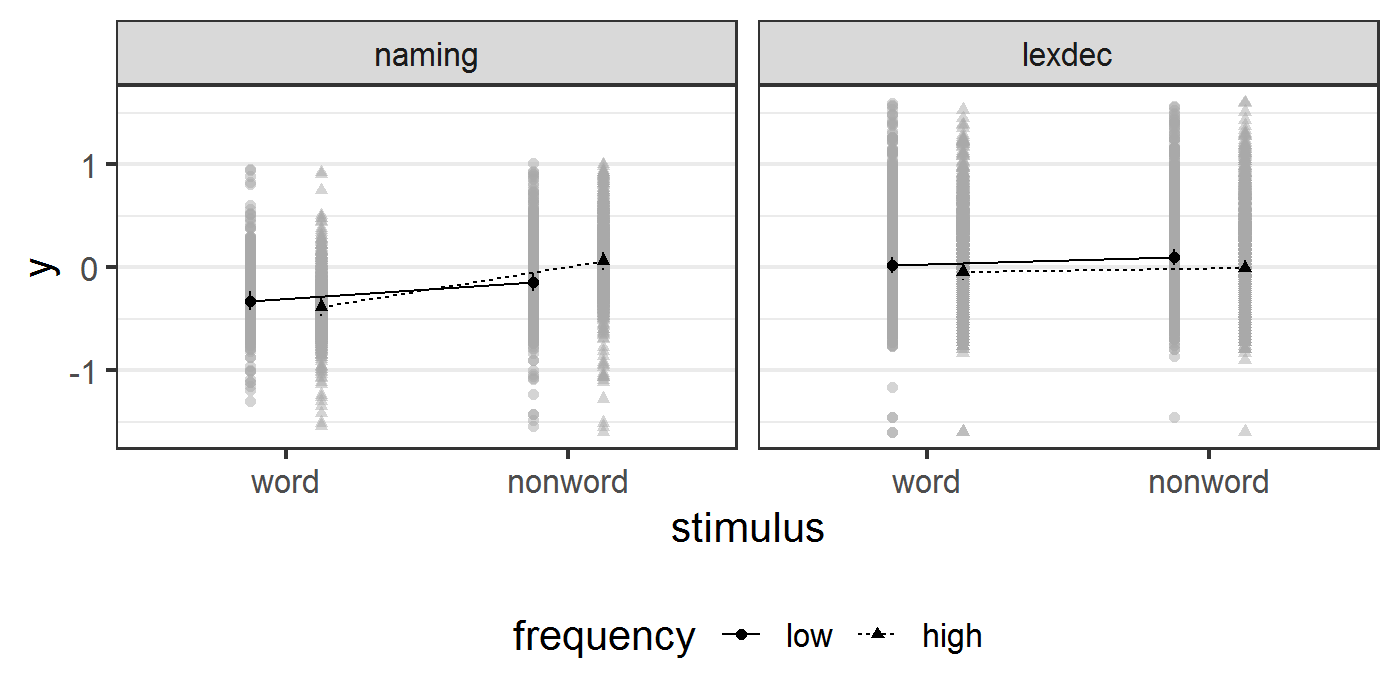In the present case, a message informs us that the data is aggregated over both random-effects grouping factors. However, this leads to way too many data points in the background. Let us compare this plot with plots in which we use each of the two random-effects grouping factors in turn.

plot_grid(
afex_plot(mrt, x = "stimulus", trace = "frequency", panel = "task",
random = "id"),
afex_plot(mrt, x = "stimulus", trace = "frequency", panel = "task",
random = "item"),
labels = c("ID", "Item")
)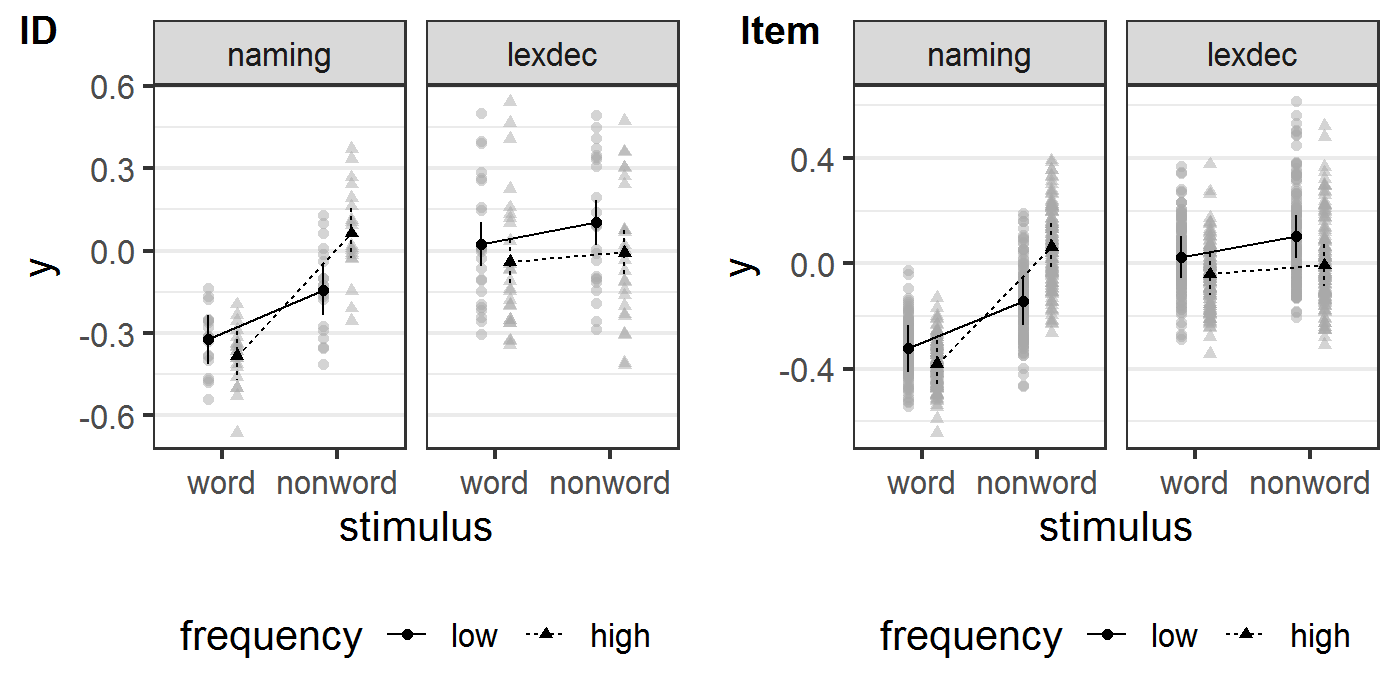The by-id plot looks usable. However, the by-item plot has still way too many data-points to be informative. Some other ways of displaying the raw data, such as violin plots or box plots, seems preferable for it.

## Ways of Plotting Data in the Background

We compare violin plots or box plots for the by-item data in the next plot. For the box plot, we increase the width of the error bars and use a consistent line type to distinguish them more easily from the graphical elements of the box plot. We could probably further improve these plots by, for example, adding colors or using some of the other customizations discussed above for the ANOVA example.

plot_grid(
afex_plot(mrt, x = "stimulus", trace = "frequency", panel = "task",
random = "item", dodge = 0.8,
data_geom = geom_violin,
data_arg = list(width = 0.5)),
afex_plot(mrt, x = "stimulus", trace = "frequency", panel = "task",
random = "item", dodge = 0.8,
data_geom = geom_boxplot,
data_arg = list(width = 0.5),
error_arg = list(size = 1.5, width = 0, linetype = 1))
)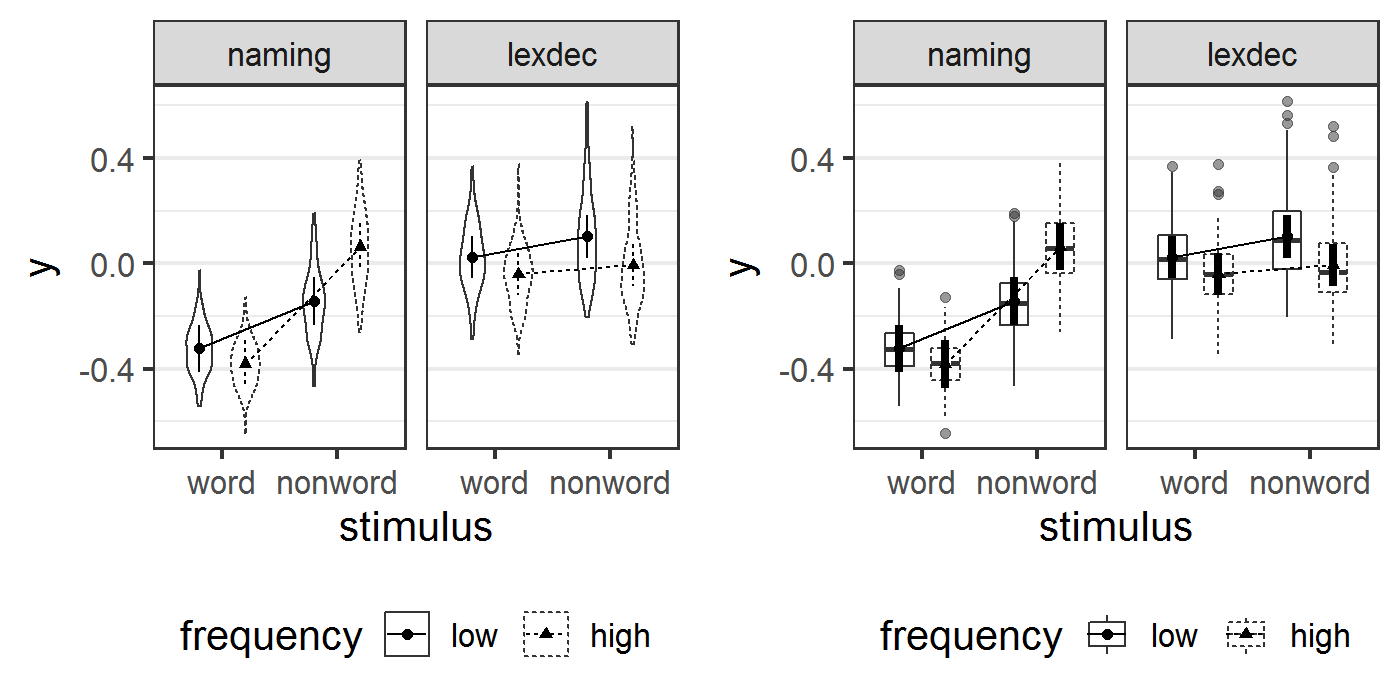## Error Bars for Mixed Models

The default error bars for afex_plot() are based on the statistical model (i.e., the mixed model in the present case). These error bars can only be used to judge whether or not two means differ from each other, if the corresponding factor (or factors) are independent samples factors (i.e., not repeated-measures factors for any of the random-effects grouping factors). Of course, in addition to this the requirement of approximately equal sample size and variance also needs to hold. In the present case, all of the factors are repeated-measures factors with respect to one of the random-effects grouping factors. Consequently, the default error bars cannot be used for “inference by eye” for any of the factors.

This is also easy to see when looking at all pairwise comparisons between means for each of the panels/tasks. This shows that for the naming task all comparisons are significant. In visual contrast with that, the two error bars for the low versus high words are overlapping very strongly.

pairs(emmeans::emmeans(mrt, c("stimulus", "frequency"), by = "task"))
## task = naming:
##  contrast                      estimate         SE  df z.ratio p.value
##  word,low - nonword,low     -0.17988046 0.02472207 Inf  -7.276  <.0001
##  word,low - word,high        0.05864672 0.01668248 Inf   3.515  0.0025
##  word,low - nonword,high    -0.38673134 0.02599454 Inf -14.877  <.0001
##  nonword,low - word,high     0.23852717 0.02598076 Inf   9.181  <.0001
##  nonword,low - nonword,high -0.20685088 0.01672999 Inf -12.364  <.0001
##  word,high - nonword,high   -0.44537805 0.02473105 Inf -18.009  <.0001
##
##  contrast                      estimate         SE  df z.ratio p.value
##  word,low - nonword,low     -0.07927031 0.02267260 Inf  -3.496  0.0027
##  word,low - word,high        0.06335085 0.01563197 Inf   4.053  0.0003
##  word,low - nonword,high     0.02986740 0.02370870 Inf   1.260  0.5887
##  nonword,low - word,high     0.14262117 0.02371722 Inf   6.013  <.0001
##  nonword,low - nonword,high  0.10913772 0.01569202 Inf   6.955  <.0001
##  word,high - nonword,high   -0.03348345 0.02254429 Inf  -1.485  0.4464
##
## P value adjustment: tukey method for comparing a family of 4 estimates

An alternative in the present situation would be using within-subjects error bars and aggregating the data by-id (i.e., error = "within"), as done in the left panel below. This is somewhat appropriate here as the factors within each panel are all within-subject factors. In contrast, using by-item within-subjects error bars, as done in the right panel below, seems not appropriate as the only within-item factor, task, is spread across panels. Unfortunately, it is not immediately clear if these error bars allow one to correctly detect, which means do not differ from each other.

plot_grid(
afex_plot(mrt, x = "stimulus", trace = "frequency", panel = "task",
random = "id", error = "within"),
afex_plot(mrt, x = "stimulus", trace = "frequency", panel = "task",
random = "item", dodge = 0.8, error = "within",
data_geom = geom_violin,
data_arg = list(width = 0.5))
)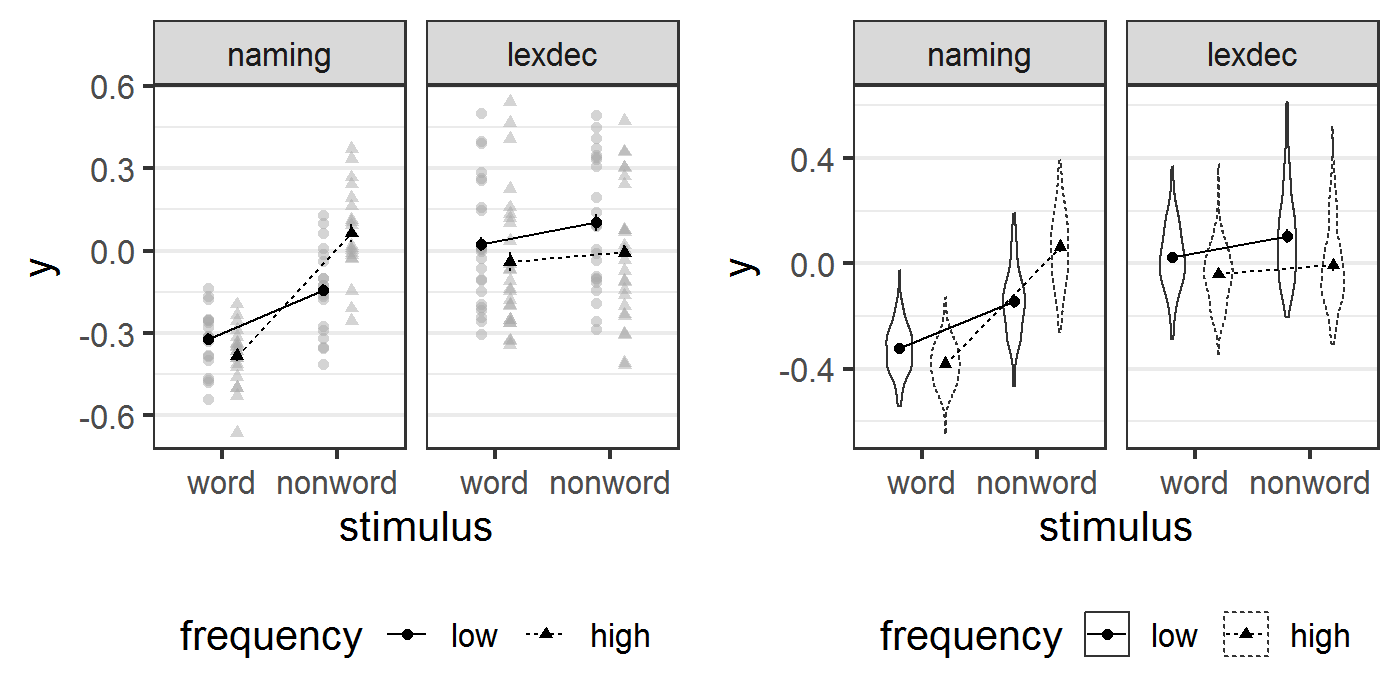In sum, using error bars for performing “inference by eye” - that is, using overlap or non-overlap of error bars to judge which means differ or do not differ from each other - is highly problematic for mixed models, due to the potentially complex dependency structures between the means. It would be best to avoid comparisons between means altogether. Instead, it is perhaps a good idea to plot the model-based error bars (which is the default) and use them for their intended purpose; judging which values of the estimated means are likely given what we have learned from the model (however, note that one cannot interpret a 95% confidence interval as having a 95% probability of containing the population mean).

The help page ?afex_plot contains further information and references on how to interpret confidence intervals and other error bars.